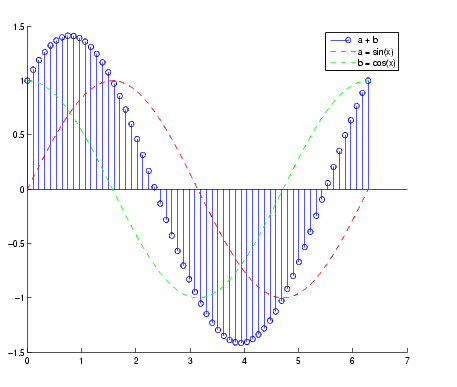GraphicsCombining Stem Plots with Line Plots

Sometimes it is useful to display more than one plot simultaneously with a stem plot to show how you arrived at a result. For example, create a linearly spaced vector with 60 elements and define two functions, `a` and `b`.

• ```x = linspace(0,2*pi,60);
a = sin(x);
b = cos(x);
```

Create a stem plot showing the linear combination of the two functions.

• ```stem_handles = stem(x,a+b);
```

Overlaying `a` and `b` as line plots helps visualize the functions. Before plotting the two curves, set `hold` to `on` so MATLAB does not clear the stem plot.

• ```hold on
plot_handles = plot(x,a,'--r',x,b,'--g');
hold off
```

Use `legend` to annotate the graph. The stem and plot handles passed to `legend` identify the lines to label. Stem plots are composed of two lines; one draws the markers and the other draws the vertical stems. To create the legend, use the first handle returned by `stem`, which identifies the marker line.

• ```legend_handles = [stem_handles(1);plot_handles];
legend(legend_handles,'a + b','a = sin(x)','b = cos(x)')
```

Labeling the axes and creating a title finishes the graph.

• ```xlabel('Time in \musecs')
ylabel('Magnitude')
title('Linear Combination of Two Functions')```Two-Dimensional Stem Plots Three-Dimensional Stem Plots© 1994-2005 The MathWorks, Inc.